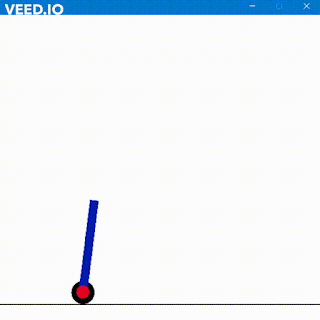### Verilog Code for Up Counters

Hola Amigos
Up Counter can be defined in a simple way don't you think ?
It just counts. HaHa

Yes!! A counter just counts up to the maximum decimal value when all the required bits are 1.
Crazy Definition  ? I agree. Let me elaborate.

Let's take an example of 3 bit counter. So whats the maximu of 3bit counter i.e when all bits are 1 in other words "111" It's 7.
Similarly for 4 bits it "1111"that is 15.

Thus n-bits we require n Flip Flops.
Thus an Up counter will count from 0 upto the maximum as I have stated ad will again start from 0.

Lets code for a 4-bit Up Counter

```module up(out,en,clk,reset);
output reg[7:0] out;
input en, clk, reset;
initial
out = 0;
always @(posedge clk)begin
if (reset) begin //if reset is true
out <= 8'b0 ;
end
else if (en==1 && out<15)  //if enable is high // because 1111 is 15
out <= out + 1;
else
out <= 0;
end

endmodule
//*************************TestBench
module uptest();
reg en;
reg clk;
reg reset;
wire [7:0]out;

initial begin
reset=0;
clk=0;
en=1;
#80 en=0;
end

up call(out,en,clk,reset);
always #2 clk=!clk;
endmodule```
```
```
```
Here is the output
```
```
Notice after 80ns output is zero because I have set en pin to 0 in testbench.

```
```
```
```
```